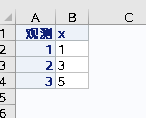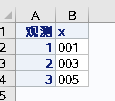## when using ods excel ,the output variable 's format is not expected

DEAR ALL：

The code as follows：

``````data test;
input x \$3.;
datalines;
001
003
005
;
run;
ods listing close ;
ods results off;
filename fbout "d:\result.xlsx";
ods excel (id=fb) file=fbout  options(sheet_interval="none" sheet_name="test");
proc print data=test;run;
ods excel (id=fb) close;
filename fbout clear;
ods listing  ;
ods results on;``````

when i open the excel file "`"d:\result.xlsx";`" ,it showsbutis what I want.

How should I correct my code in sas, not in excel ？

4 REPLIES 4

## Re: when using ods excel ,the output variable 's format is not expected

Maybe there are simpler methods, but you can add ASCII 255 in front of your zeros, this unprintable character will force Excel to see the character string as character and not numeric.

``````data test1;
length x \$ 4;
set test;
x=cat(' ',x);
run;
ods excel file="test.xlsx";
proc print data=test1;
run;
ods excel close;``````

In the CAT function inside the quotes is not a blank, even though it looks like a blank. It is ASCII 255, which you can type by holding down the Alt key, pressing 2 5 5 on the numeric keypad and then letting go of the Alt key. Of course, this prevents the numbers from being treated as numbers in Excel, which may (or may not) be a problem for you.

--
Paige Miller

## Re: when using ods excel ,the output variable 's format is not expected

You should search this forum to answers as this is a common topic.

The easiest way to fix this is use the XLSX libref engine to just dump the data in Excel instead of trying to use ODS EXCEL to store a report into Excel.

``````filename fbout "d:\result.xlsx";
ods excel (id=fb) file=fbout  options(sheet_interval="none" sheet_name="test");
proc print data=test;run;
ods excel (id=fb) close;``````

Use

``````libname fbout xlsx "d:\result.xlsx";
data fbout.test;
set test;
run;
libname fbout close;``````

To fix the ODS output you will need to look into how to use style options to set the TAGATTR attribute to let Excel know the cells the column created for that variable should be treated as TEXT and not GENERAL.

## Re: when using ods excel ,the output variable 's format is not expected

You might also consider Proc Report as an alternative where STYLE attributes forces excel to print values in desired format.
Eg. the below code prints leading zeros:

``````proc report data=test;
column _all_;
define x/display style(column)={tagattr='format:000'};
run;``````

## Re: when using ods excel ,the output variable 's format is not expected

Thanks all dears:

I find a surprisingly simple way to solve the problem, just add "format x \$3.;" after" proc print;"!

``````data test;
input x \$3.;
datalines;
001
003
005
;
run;
ods listing close ;
ods results off;
filename fbout "d:\result.xlsx";
ods excel (id=fb) file=fbout  options(sheet_interval="none" sheet_name="test");
proc print data=test;format x \$3.;run;
ods excel (id=fb) close;
filename fbout clear;
ods listing  ;
ods results on;``````

Discussion stats
• 4 replies
• 220 views
• 2 likes
• 4 in conversation